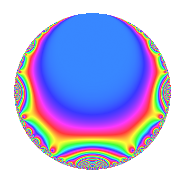# Properties

 Label 574.2.a.kLevel 574 Weight 2 Character orbit 574.a Self dual Yes Analytic conductor 4.583 Analytic rank 0 Dimension 2 CM No Inner twists 1

# Related objects

## Newspace parameters

 Level: $$N$$ = $$574 = 2 \cdot 7 \cdot 41$$ Weight: $$k$$ = $$2$$ Character orbit: $$[\chi]$$ = 574.a (trivial)

## Newform invariants

 Self dual: Yes Analytic conductor: $$4.58341307602$$ Analytic rank: $$0$$ Dimension: $$2$$ Coefficient field: $$\Q(\sqrt{3})$$ Coefficient ring: $$\Z[a_1, \ldots, a_{5}]$$ Coefficient ring index: $$1$$ Fricke sign: $$-1$$ Sato-Tate group: $\mathrm{SU}(2)$

## $q$-expansion

Coefficients of the $$q$$-expansion are expressed in terms of $$\beta = \sqrt{3}$$. We also show the integral $$q$$-expansion of the trace form.

 $$f(q)$$ $$=$$ $$q + q^{2} + 2 q^{3} + q^{4} + ( 1 + \beta ) q^{5} + 2 q^{6} + q^{7} + q^{8} + q^{9} +O(q^{10})$$ $$q + q^{2} + 2 q^{3} + q^{4} + ( 1 + \beta ) q^{5} + 2 q^{6} + q^{7} + q^{8} + q^{9} + ( 1 + \beta ) q^{10} + ( -1 - \beta ) q^{11} + 2 q^{12} + ( -2 - 2 \beta ) q^{13} + q^{14} + ( 2 + 2 \beta ) q^{15} + q^{16} + q^{18} -2 \beta q^{19} + ( 1 + \beta ) q^{20} + 2 q^{21} + ( -1 - \beta ) q^{22} -2 q^{23} + 2 q^{24} + ( -1 + 2 \beta ) q^{25} + ( -2 - 2 \beta ) q^{26} -4 q^{27} + q^{28} + ( 1 - 5 \beta ) q^{29} + ( 2 + 2 \beta ) q^{30} + ( -2 + 2 \beta ) q^{31} + q^{32} + ( -2 - 2 \beta ) q^{33} + ( 1 + \beta ) q^{35} + q^{36} + ( -2 + 4 \beta ) q^{37} -2 \beta q^{38} + ( -4 - 4 \beta ) q^{39} + ( 1 + \beta ) q^{40} + q^{41} + 2 q^{42} + ( 2 + 6 \beta ) q^{43} + ( -1 - \beta ) q^{44} + ( 1 + \beta ) q^{45} -2 q^{46} + ( 4 - 2 \beta ) q^{47} + 2 q^{48} + q^{49} + ( -1 + 2 \beta ) q^{50} + ( -2 - 2 \beta ) q^{52} + ( -1 + 5 \beta ) q^{53} -4 q^{54} + ( -4 - 2 \beta ) q^{55} + q^{56} -4 \beta q^{57} + ( 1 - 5 \beta ) q^{58} + ( 1 + 7 \beta ) q^{59} + ( 2 + 2 \beta ) q^{60} + ( 5 + \beta ) q^{61} + ( -2 + 2 \beta ) q^{62} + q^{63} + q^{64} + ( -8 - 4 \beta ) q^{65} + ( -2 - 2 \beta ) q^{66} + ( -5 + 3 \beta ) q^{67} -4 q^{69} + ( 1 + \beta ) q^{70} + ( 6 - 2 \beta ) q^{71} + q^{72} + ( -2 - 4 \beta ) q^{73} + ( -2 + 4 \beta ) q^{74} + ( -2 + 4 \beta ) q^{75} -2 \beta q^{76} + ( -1 - \beta ) q^{77} + ( -4 - 4 \beta ) q^{78} + ( 1 + \beta ) q^{80} -11 q^{81} + q^{82} + ( 3 + \beta ) q^{83} + 2 q^{84} + ( 2 + 6 \beta ) q^{86} + ( 2 - 10 \beta ) q^{87} + ( -1 - \beta ) q^{88} + ( 6 + 4 \beta ) q^{89} + ( 1 + \beta ) q^{90} + ( -2 - 2 \beta ) q^{91} -2 q^{92} + ( -4 + 4 \beta ) q^{93} + ( 4 - 2 \beta ) q^{94} + ( -6 - 2 \beta ) q^{95} + 2 q^{96} + 2 q^{97} + q^{98} + ( -1 - \beta ) q^{99} +O(q^{100})$$ $$\operatorname{Tr}(f)(q)$$ $$=$$ $$2q + 2q^{2} + 4q^{3} + 2q^{4} + 2q^{5} + 4q^{6} + 2q^{7} + 2q^{8} + 2q^{9} + O(q^{10})$$ $$2q + 2q^{2} + 4q^{3} + 2q^{4} + 2q^{5} + 4q^{6} + 2q^{7} + 2q^{8} + 2q^{9} + 2q^{10} - 2q^{11} + 4q^{12} - 4q^{13} + 2q^{14} + 4q^{15} + 2q^{16} + 2q^{18} + 2q^{20} + 4q^{21} - 2q^{22} - 4q^{23} + 4q^{24} - 2q^{25} - 4q^{26} - 8q^{27} + 2q^{28} + 2q^{29} + 4q^{30} - 4q^{31} + 2q^{32} - 4q^{33} + 2q^{35} + 2q^{36} - 4q^{37} - 8q^{39} + 2q^{40} + 2q^{41} + 4q^{42} + 4q^{43} - 2q^{44} + 2q^{45} - 4q^{46} + 8q^{47} + 4q^{48} + 2q^{49} - 2q^{50} - 4q^{52} - 2q^{53} - 8q^{54} - 8q^{55} + 2q^{56} + 2q^{58} + 2q^{59} + 4q^{60} + 10q^{61} - 4q^{62} + 2q^{63} + 2q^{64} - 16q^{65} - 4q^{66} - 10q^{67} - 8q^{69} + 2q^{70} + 12q^{71} + 2q^{72} - 4q^{73} - 4q^{74} - 4q^{75} - 2q^{77} - 8q^{78} + 2q^{80} - 22q^{81} + 2q^{82} + 6q^{83} + 4q^{84} + 4q^{86} + 4q^{87} - 2q^{88} + 12q^{89} + 2q^{90} - 4q^{91} - 4q^{92} - 8q^{93} + 8q^{94} - 12q^{95} + 4q^{96} + 4q^{97} + 2q^{98} - 2q^{99} + O(q^{100})$$

## Embeddings

For each embedding $$\iota_m$$ of the coefficient field, the values $$\iota_m(a_n)$$ are shown below.

For more information on an embedded modular form you can click on its label.

Label $$\iota_m(\nu)$$ $$a_{2}$$ $$a_{3}$$ $$a_{4}$$ $$a_{5}$$ $$a_{6}$$ $$a_{7}$$ $$a_{8}$$ $$a_{9}$$ $$a_{10}$$
1.1
 −1.73205 1.73205
1.00000 2.00000 1.00000 −0.732051 2.00000 1.00000 1.00000 1.00000 −0.732051
1.2 1.00000 2.00000 1.00000 2.73205 2.00000 1.00000 1.00000 1.00000 2.73205
 $$n$$: e.g. 2-40 or 990-1000 Significant digits: Format: Complex embeddings Normalized embeddings Satake parameters Satake angles

## Inner twists

This newform does not admit any (nontrivial) inner twists.

## Atkin-Lehner signs

$$p$$ Sign
$$2$$ $$-1$$
$$7$$ $$-1$$
$$41$$ $$-1$$

## Hecke kernels

This newform can be constructed as the intersection of the kernels of the following linear operators acting on $$S_{2}^{\mathrm{new}}(\Gamma_0(574))$$:

 $$T_{3} - 2$$ $$T_{5}^{2} - 2 T_{5} - 2$$ $$T_{11}^{2} + 2 T_{11} - 2$$